# Direct product of D8 and Z2

(diff) ← Older revision | Latest revision (diff) | Newer revision → (diff)
View a complete list of particular groups (this is a very huge list!)[SHOW MORE]

## Definition

This group is defined in the following ways:

A presentation for it is:$G := \langle a,x,y \mid a^4 = x^2 = y^2 = e, xax = a^{-1}, ya = ay, xy = yx \rangle$.

## Elements

### Upto conjugacy

There are ten conjugacy classes:

1. The identity element. (1)
2. The element$a^2$. (1)
3. The element$y$. (1)
4. The element$a^2y$. (1)
5. The two-element conjugacy class comprising$a$ and$a^3$. (2)
6. The two-element conjugacy class comprising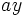$ay$ and$a^3y$. (2)
7. The two-element conjugacy class comprising$x$ and$a^2x$. (2)
8. The two-element conjugacy class comprising$xy$ and$a^2xy$. (2)
9. The two-element conjugacy class comprising$ax$ and$a^3x$. (2)
10. The two-element conjugacy class comprising$axy$ and$a^3xy$. (2)

### Upto automorphism

Under the action of the automorphism group, the conjugacy classes (3) and (4) are in the same orbit -- in other words,$y$ and$a^2y$ are related by an automorphism. The conjugacy classes (5) and (6) are equivalent, and the conjugacy classes (7)-(10) are equivalent. Thus, the equivalence classes have sizes$1,1,2,4,8$.

## Arithmetic functions

Want to compare and contrast arithmetic function values with other groups of the same order? Check out groups of order 16#Arithmetic functions
Function Value Similar groups Explanation for function value
underlying prime of p-group 2
order (number of elements, equivalently, cardinality or size of underlying set) 16 groups with same order The group is an external direct product of a group (dihedral group:D8) of order 8 and a group (cyclic group:Z2) of order 2, and order of direct product is product of orders
prime-base logarithm of order 4 groups with same prime-base logarithm of order
max-length of a group 4 max-length of a group equals prime-base logarithm of order for group of prime power order
chief length 4 chief length equals prime-base logarithm of order for group of prime power order
composition length 4 composition length equals prime-base logarithm of order for group of prime power order
exponent of a group 4 groups with same order and exponent of a group | groups with same exponent of a group The group is an external direct product of a group (dihedral group:D8) of exponent 4 and a group (cyclic group:Z2) of exponent 2, and exponent of direct product is lcm of exponents
prime-base logarithm of exponent 2 groups with same order and prime-base logarithm of exponent | groups with same prime-base logarithm of order and prime-base logarithm of exponent | groups with same prime-base logarithm of exponent
nilpotency class 2 groups with same order and nilpotency class | groups with same prime-base logarithm of order and nilpotency class | groups with same nilpotency class
derived length 2 groups with same order and derived length | groups with same prime-base logarithm of order and derived length | groups with same derived length
Frattini length 2 groups with same order and Frattini length | groups with same prime-base logarithm of order and Frattini length | groups with same Frattini length
Fitting length 1 The group is a nilpotent group, hence its Fitting length is 1. Note that prime power order implies nilpotent, so all groups of the same order have Fitting length 1.
minimum size of generating set 3 groups with same order and minimum size of generating set | groups with same prime-base logarithm of order and minimum size of generating set | groups with same minimum size of generating set Generating set of dihedral direct factor and generating element for other direct factor (also equal to rank of Frattini quotient).
subgroup rank of a group 3 groups with same order and subgroup rank of a group | groups with same prime-base logarithm of order and subgroup rank of a group | groups with same subgroup rank of a group
rank of a p-group 3 groups with same order and rank of a p-group | groups with same prime-base logarithm of order and rank of a p-group | groups with same rank of a p-group Direct product of Klein four-subgroup of first direct factor with second direct factor.
normal rank of a p-group 3 groups with same order and normal rank of a p-group | groups with same prime-base logarithm of order and normal rank of a p-group | groups with same normal rank of a p-group Direct product of Klein four-subgroup of first direct factor with second direct factor.
characteristic rank of a p-group 2 groups with same order and characteristic rank of a p-group | groups with same prime-base logarithm of order and characteristic rank of a p-group | groups with same characteristic rank of a p-group Center is a Klein four-subgroup, no characteristic elementary abelian subgroups of order eight

## Group properties

Property Satisfied? Explanation Corollary properties satisfied/dissatisfied
abelian group No$a$ and$x$ don't commute Dissatisfies: cyclic group
group of prime power order Yes Prime power order implies nilpotent Satisfies: nilpotent group (prime power order implies nilpotent), supersolvable group (finite nilpotent implies supersolvable), solvable group (nilpotent implies solvable)
metacyclic group No
T-group No$\langle x \rangle \triangleleft \langle a^2,x \rangle$, which is normal, but$\langle x \rangle$ is not normal. See also normality is not transitive
monolithic group No Center is Klein four-group, any subgroup of order two is minimal normal.
one-headed group No Distinct maximal subgroups of order eight.
SC-group No
ACIC-group Yes Every automorph-conjugate subgroup is characteristic
rational-representation group Yes All representations over characteristic zero are realized over the rationals. Satisfies: rational group, ambivalent group
directly indecomposable group No Dissatisfies: splitting-simple group, centrally indecomposable group
UL-equivalent group No It is a direct product of groups of different nilpotency class values. See also nilpotent not implies UL-equivalent

## Subgroups

Further information: Subgroup structure of direct product of D8 and Z2

The group has the following subgroups:

1. The trivial group. (1)
2. The cyclic group$\langle a^2 \rangle$ of order two. This is central, and is also the set of squares. Isomorphic to cyclic group:Z2. (1)
3. The subgroups$\langle y \rangle$ and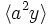$\langle a^2y \rangle$. These are both central subgroups of order two, but are related by an outer automorphism. Isomorphic to cyclic group:Z2. (2)
4. The subgroups$\langle x \rangle$,$\langle ax \rangle$,$\langle a^2x \rangle$,$\langle a^3x \rangle$,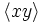$\langle xy \rangle$,$\langle axy \rangle$,$\langle a^2xy \rangle$, and$\langle a^3xy \rangle$. These are all related by automorphisms, and are all 2-subnormal subgroups. They come in four conjugacy classes, namely the class$\langle x \rangle, \langle a^2x \rangle$, the class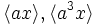$\langle ax \rangle, \langle a^3x \rangle$, the class$\langle xy \rangle, \langle a^2xy$, and the class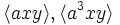$\langle axy \rangle, \langle a^3xy \rangle$. Isomorphic to cyclic group:Z2. (8)
5. The subgroup$\langle a^2, y \rangle$. This is the center, hence is a characteristic subgroup. Isomorphic to Klein four-group. (1)
6. The subgroups$\langle a^2, x \rangle$,$\langle a^2, ax \rangle$,$\langle a^2, xy \rangle$, and$\langle a^2, axy \rangle$. These are all normal subgroups, but are related by outer automorphisms. Isomorphic to Klein four-group. (4)
7. The subgroups$\langle y, x \rangle$,$\langle y, ax \rangle$,$\langle y, a^2x \rangle$,$\langle y, a^3x$,$\langle a^2y, x \rangle$,$\langle a^2y, ax$,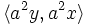$\langle a^2y, a^2x \rangle$,$\langle a^2y, a^3x \rangle$. These subgroups are all 2-subnormal subgroups and are related by outer automorphisms, and they come in four conjugacy classes of size two. Isomorphic to Klein four-group. (8)
8. The subgroups$\langle a \rangle$ and$\langle ay \rangle$. They are all normal and are related via outer automorphisms. Isomorphic to cyclic group:Z4. (2)
9. The subgroups$\langle a^2,x,y \rangle$ and$\langle a^2, ax, y \rangle$. These are normal and are related by outer automorphisms. Isomorphic to elementary abelian group of order eight. (2)
10. The subgroups$\langle a,x \rangle$,$\langle a, xy \rangle$,$\langle ay, x \rangle$ and$\langle ay, xy \rangle$. These are both normal and are related by an outer automorphism. Isomorphic to dihedral group:D8. (4)
11. The subgroup$\langle a, y \rangle$. This is a characteristic subgroup. Isomorphic to direct product of Z4 and Z2. (1)
12. The whole group. (1)

## GAP implementation

### Group ID

This finite group has order 16 and has ID 11 among the groups of order 16 in GAP's SmallGroup library. For context, there are 14 groups of order 16. It can thus be defined using GAP's SmallGroup function as:

SmallGroup(16,11)

For instance, we can use the following assignment in GAP to create the group and name it$G$:

gap> G := SmallGroup(16,11);

Conversely, to check whether a given group$G$ is in fact the group we want, we can use GAP's IdGroup function:

IdGroup(G) = [16,11]

or just do:

IdGroup(G)

to have GAP output the group ID, that we can then compare to what we want.

### Hall-Senior number

This group of prime power order has order 16 and has Hall-Senior number 6 among the groups of order 16. This information can be used to construct the group in GAP using the Gap3CatalogueGroup function as follows:

Gap3CatalogueGroup(16,6)

WARNING: There is some disagreement between the GAP 3 catalogue numbers and the Hall-Senior numbers for some abelian groups, but it does not affect this group.

For instance, we can use the following assignment in GAP to create the group and name it$G$:

gap> G := Gap3CatalogueGroup(16,6);

Conversely, to check whether a given group$G$ is in fact the group we want, we can use GAP's Gap3CatalogueIdGroup function:

Gap3CatalogueIdGroup(G) = [16,6]

or just do:

Gap3CatalogueIdGroup(G)

to have GAP output the group ID, that we can then compare to what we want.

### Other descriptions

Description Functions used
DirectProduct(DihedralGroup(8),CyclicGroup(2)) DirectProduct, DihedralGroup, CyclicGroup
SylowSubgroup(SymmetricGroup(6),2) SylowSubgroup, SymmetricGroup